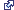# Working with NumPy in ArcGIS

Numerical Python (NumPy) is a fundamental package for scientific computing in Python, including support for a powerful N-dimensional array object. NumPy allows you to perform complex mathematical operations and has been part of the ArcGIS software installation since 9.2. For more information, see the NumPy website.

A Python NumPy array is designed to work with large arrays. There are many existing Python functions that have been created to process NumPy arrays, the most noted being contained in the SciPy scientific computing package for Python.

## Working with tables and feature data

Table and feature classes can be converted to and from NumPy arrays using functions in the data access (arcpy.da) module.

To convert NumPy arrays to tables and feature classes, the arrays must be structured arrays. Structured arrays include fields (or structs) that are used to map the data to field in ArcGIS table and feature classes. For more information on structured arrays, see Structured arrays.

Create a structured NumPy array.

``````import numpy

arr = numpy.array([(471316.383, 5000448.782), (470402.493, 5000049.216)],
numpy.dtype([('X', '>f8'),('Y', '>f8')]))``````

Once created, a structured NumPy array can be converted to a feature class or table.

Convert a NumPy array to a geodatabase feature class.

``````import arcpy
import numpy

out_fc = 'C:/data/texas.gdb/fd/pointlocations'

# Create a numpy array with an id field, and a field with a tuple
#  of x,y coordinates
arr = numpy.array([(1, (471316.3835861763, 5000448.782036674)),
(2, (470402.49348005146, 5000049.216449278))],
numpy.dtype([('idfield', numpy.int32),('XY', '<f8', 2)]))

# Define a spatial reference for the output feature class
spatial_ref = arcpy.Describe('C:/data/texas.gdb/fd').spatialReference

# Export the numpy array to a feature class using the XY field to
#  represent the output point feature
arcpy.da.NumPyArrayToFeatureClass(arr, out_fc, ['XY'], spatial_ref)``````

#### arcpy.da functions for working with tables and feature data

FunctionExplanation
ExtendTable

Join the contents of a NumPy structured array to a table based on a common attribute field.

FeatureClassToNumPyArray

Convert a feature class to a NumPy structured array.

NumPyArrayToFeatureClass

Convert a NumPy structured array to a feature class.

NumPyArrayToTable

Convert a NumPy structured array to a table.

TableToNumPyArray

Convert a table to a NumPy structured array.

Integer fields in NumPy arrays do not support nulls. If data converted using FeatureClassToNumPyArray or TableToNumPyArray contains nulls, the rows containing the nulls should either be skipped entirely or masked with a substitute value.

Skip all records that include a null.

``arr = arcpy.da.FeatureClassToNumPyArray(fc, fields, skip_nulls=True)``

Mask each None in integer fields with different values using a dictionary.

``````fields = ['field1', 'field2']
arcpy.da.FeatureClassToNumPyArray(fc, fields, null_value=-9999)``````

### Type conversions

The dtypes of the created array are determined from the field type of the input table or feature class.

Field typeNumPy dtype

Single

numpy.float32

Double

numpy.float64

SmallInteger

numpy.int32

Integer

numpy.int32

OID

numpy.int32

GUID

<U64

String

<u1, <u10, and so on

Date

<M8[us]

##### Note:

String fields converted to an array will have the same width. For instance, a string field with a width of 20 will have a dtype of <u20.

Other field types not listed above, including raster and BLOB fields, are not supported. Geometry fields are also not supported, but multiple geometry properties can be added to the array using the special tokens listed below.

TokenDescription

SHAPE@XY

A tuple of the feature's centroid x,y coordinates.

SHAPE@XYZ

A tuple of the feature's centroid x,y,z coordinates.

SHAPE@TRUECENTROID

A tuple of the feature's centroid x,y coordinates. This returns the same value as SHAPE@XY.

SHAPE@X

A double of the feature's x-coordinate.

SHAPE@Y

A double of the feature's y-coordinate.

SHAPE@Z

A double of the feature's z-coordinate.

SHAPE@M

A double of the feature's m-value.

SHAPE@JSON

The Esri JSON string representing the geometry.

SHAPE@WKB

The well-known binary (WKB) representation for OGC geometry. It provides a portable representation of a geometry value as a contiguous stream of bytes.

SHAPE@WKT

The well-known text (WKT) representation for OGC geometry. It provides a portable representation of a geometry value as a text string.

SHAPE@AREA

A double of the feature's area.

SHAPE@LENGTH

A double of the feature's length.

OID@

The value of the Object ID field.

### Memory considerations

An array that requires more memory than is available will fail with a MemoryError exception.

The following are tips to avoid MemoryError exceptions:

• Delete array objects after use; deleting the array will release the memory.
• Use only those fields you need, especially text fields; a text field converted to an array will consume 4 bytes for every character of width. For instance, a string field with a width of 100 will consume 400 bytes of memory for each value in the array.
NumPy dtypeNumber of bytes per value

<U1

4

numpy.int32

4

numpy.float32

4

numpy.float64

8

##### Note:

numpy.nbytes returns a dictionary of dtypes and number of bytes.

## Working with rasters

Rasters can be converted to and from NumPy arrays using the ArcPy functions RasterToNumPyArray and NumPyArrayToRaster. You may want to convert an ArcGIS raster to a NumPy array to do the following:

• Implement one of the many existing Python functions that can be applied to a NumPy array (for example, run filters on the data, perform multidimensional analysis, or use optimization routines).
• Develop a custom function by accessing the individual cells within the NumPy array (for example, to implement neighborhood notation, change individual cell values, or run accumulative operators on an entire raster).

#### arcpy functions for working with rasters

FunctionExplanation
RasterToNumPyArray

Convert a raster to a NumPy array.

NumPyArrayToRaster

Convert a NumPy array to a raster.

A raster is converted to a NumPy array to calculate the percentage of the cell value in the entire raster row. A new raster is then created.

``````import arcpy
import numpy

my_array = arcpy.RasterToNumPyArray('C:/data/inRaster')
my_array_sum = my_array.sum(1)
my_array_sum.shape = (my_array.shape, 1)
my_array_perc = (my_array * 1.0) / my_array_sum

new_raster = arcpy.NumPyArrayToRaster(my_array_perc)
new_raster.save("C:/output/fgdb.gdb/PercentRaster")``````